# Mating type switching in natural isolates

This file contains scripts and information for the manuscript:

“Self-compatibility in yeast is selected for reproductive assurance not population level compatibility”

# Index

Note: R code was run locally using Rstudio

Bash scripts were run either on the BioHPC cluster at LRZ, or on a local cluster (corvus) at the Faculty of Biology of LMU.

# Empirical and bioinformatics

## Pipeline to quantify P and M in genomic data

1. map reads to both h90_P and to h90_M artificial reference genomes using bwa .
2. sort and index using samtools
3. get coverage for entire genome
4. cut down to contain MTR chromosome and region chrII:2113093-2133503
5. obtain all read IDs that map to the RTS1 region (359bp from H2 towards centromere)
6. check if the RTS1 regions seems to by unique by comparing coverage to the mean coverage of the strain
7. obtain those reads and their mate pairs
8. transform to fastq
9. use bbsplit to map reads simultaneously to either of two mating type reference fasta files which results in 3 files (one for P, one for M and one for ambiguous)
10. count lines in fastq files / 4 for number of reads in either strain

In the folder `/data/home/wolfproj/wolfproj-13/switching/analyses/` we get all the files we want to analyze and we split these into 5 separate batches to run in parallel:

``````for F in /home/wolfproj/tmp/Bart/pombe/natural_isolates/fastq/*_1.fastq.gz; \
do base=\${F##*/}; \
echo \${base/_1.fastq.gz/} >> files.txt; \
done
wc -l files.txt # returns 357 so we need 357/5=71 --> 71+1 lines per file
split --lines=72 files.txt``````

Then run the script below using:

``for batch in x*; do sbatch ../scripts/pipeline.sh \$batch; done``

The script below (corvus cluster) takes in as a parameter the file name containing the file names without the information of the paired end and file type information of the fastq files as generated above.

``````#!/bin/bash
#SBATCH --job-name="map_P&M"
#SBATCH --mem=8000
#SBATCH --mail-user=nieuwenhuis@bio.lmu.de
#SBATCH --mail-type=end
#SBATCH --time=2-00:00:00

INP=\$1

## We will be mapping all reads both to an h90 reference genome with a
## plus cassette at the mat1 locus and with a minus cassette at this locus,
## to avoid bias from using one or the other as a reference. These references
## do not contain the mating type "chromosome" MTR from the reference genomes.
## These are references as described in Nieuwenhuis, Tusso et al. 2018.
#bwa index ../references/h90_full_M.fasta
#bwa index ../references/h90_full_P.fasta

DIRIN="/data/home/wolfproj/tmp/Bart/pombe/natural_isolates/fastq/"

## most intermediate files are remove, but some we want to keep
## to avoid having to repeat everything again...

# mkdir -p fastq # to store the to be mapped fastq files
# mkdir -p coverages # to store coverage info files
# mkdir -p assigned_reads # to store the mat2P or mat3M reads
# mkdir -p short_samfiles # to store the samfile with reads in the mat23 region

## map to references
bwa mem ../references/h90_full_M.fasta \$DIRIN/\${f}_1.fastq.gz \$DIRIN/\${f}_2.fastq.gz > \${f}_M.sam
bwa mem ../references/h90_full_P.fasta \$DIRIN/\${f}_1.fastq.gz \$DIRIN/\${f}_2.fastq.gz > \${f}_P.sam

## change to bam
samtools view -bS \${f}_M.sam > \${f}_M.bam && rm \${f}_M.sam
samtools view -bS \${f}_P.sam > \${f}_P.bam && rm \${f}_P.sam

## sort and index bamfile
samtools sort \${f}_M.bam -o \${f}_M_sort.bam && rm \${f}_M.bam
samtools sort \${f}_P.bam -o \${f}_P_sort.bam && rm \${f}_P.bam
samtools index \${f}_M_sort.bam
samtools index \${f}_P_sort.bam

## remove intermediate files
#rm \${f}_M.sam \${f}_M.bam \${f}_P.sam \${f}_P.bam

## get coverage of the region upstream of the mating type
samtools depth -aa -r II:2000000-2100000 \${f}_M_sort.bam > \${f}_M.coverage
samtools depth -aa -r II:2000000-2100000 \${f}_P_sort.bam > \${f}_P.coverage

## get coverage of the region right before the mating type
samtools depth -aa -r II:2113449-2114449 \${f}_M_sort.bam > \${f}_M_RTS1.coverage
samtools depth -aa -r II:2113449-2114449 \${f}_P_sort.bam > \${f}_P_RTS1.coverage

#cut down bam file to chrII sub region for faster grepping of readIDs
samtools view -h \${f}_M_sort.bam "II:2113000-2133503" > \${f}_mergedPM.sam
samtools view -h \${f}_P_sort.bam "II:2113000-2133503" >> \${f}_mergedPM.sam

#extract readIDs into text file by RTS1 region IDs
samtools view -f 0x1 \${f}_M_sort.bam "II:2113093-2114093" | cut -f1 > \${f}_readID.txt
samtools view -f 0x1 \${f}_P_sort.bam "II:2113093-2114093" | cut -f1 >> \${f}_readID.txt

## make merged samfile with all potential reads of interest
# first get header from samfile (first 6 lines, doesn't matter P or M)
# to create emtpy sam file to put grepped reads into
grep '^@' -m6 \${f}_mergedPM.sam > \${f}_mat1.sam
# then grep mate pairs of RTS1 reads (use LC_ALL=C to speed up grep)
LC_ALL=C grep -w -F -f \${f}_uniq_readID.txt < \${f}_mergedPM.sam >> \${f}_mat1.sam

## Get the reads as fastq
# convert sam to bam
samtools view -bS \${f}_mat1.sam > \${f}_mat1.bam && rm \${f}_mat1.sam
# then extract the reads to obtain fastq in a paired and interleaved format
bedtools bamtofastq -i \${f}_mat1.bam -fq \${f}_mat1.fastq

## and cleanup again
rm \${f}*.bai \${f}*.bam
mv \${f}_mergedPM.sam short_samfiles
mv \${f}*.coverage coverages

# split reads into fastq files with reads that match better to 1) mat1 region where the
# mating type genes are masked by NNN or 2) a fasta file with the Plus and Minus genes.
# "int=t" means that the input file is paired and interleaved
# "outu" indicates the name for the unmapped reads.
bbsplit.sh \
in=\${f}_mat1.fastq \
ref=../references/mat2P3M.fasta,../references/aroundMat1_N.fasta \
ambiguous=toss ambiguous2=toss \
basename=\${f}_out_%.fastq \
outu=\${f}-clean.fastq \
int=t

rm \${f}-clean.fastq \${f}_out_aroundMat1_N.fastq

# split reads into fastq files with reads that better match either mat2P or mat3M
# a third file with ambiguous (equally mapping) or non-mapping reads is also generated.
# "int=t" means that the input file is paired and interleaved
# "outu" indicates the name for the unmapped reads.
bbsplit.sh \
in=\${f}_mat2P3M.fastq \
ref=../references/mat2P.fasta,../references/mat3M.fasta \
ambiguous=toss ambiguous2=toss \
basename=\${f}_out_2_%.fastq \
outu=\${f}-clean.fastq \
int=t

## and cleanup again
rm \${f}-clean.fastq \${f}_out_mat2P3M.fastq

done < \$INP

for F in *.fastq; do
awk '{} END { if(NR>0) print FILENAME "," NR/4; else print FILENAME ",0" }' \$F >> ../211112reads_per_strain.csv
done``````

### Measure frequencies for each strain

``````# when all individual files are generated, we can analyse the reads per file using this script
# read IDs always start either with @ST or with @ERR
for F in *.fastq; do
awk '\$1 ~/^@[SE][RT]/{seen[\$0]++} END {print FILENAME "," length(seen)}' \$F >> 220208uniqreads_per_strain.csv
done``````

These data are now further analysed in R (packages loaded: `library(tidyverse)` and `library(patchwork)`)

First the data is read in, and a \(chi^2\) is performed for each strain to find deviations from 1:1 (for only mat1 and for all loci). The results are then corrected for multiple testing using False Discovery Rate correction.

``````illreads_raw <-
## this file contains all the counts of the assigned number of reads
## assigned either to P or M.
## the file contains data for:
##  - M reads at mat1 : out_mat3M
##  - P reads at mat1 : out_mat2P
##  - M reads at mat1 : out2_mat3M Double bbsplit filtered
##  - P reads at mat1 : out2_mat2P Double bbsplit filtered
##  - M reads in the entire library : outAll_mat3M
##  - P reads in the entire library : outAll_mat2P
##  - M reads in the entire library : outAll2_mat3M Double bbsplit filtered
##  - P reads in the entire library : outAll2_mat2P Double bbsplit filtered
## It contains data both for the Jeffares et al. 2015 and
## for Tusso et al. 2019 (EBC strains)

# separate the file names into informative columns and remove uninfomative elements
separate( X1, into= c( "strain", "accession", "measure", "mat","extension" ) ) %>%
filter( accession != "ERR499321" ) %>% ## JB22 strain that is something else...
select(-extension) %>%
mutate( measure = as_factor( measure ))

# modify the columns to distinguish Jeffares strains from Tusso strains
mutate( EBCorJB = ifelse( str_detect(accession, "EBC" ),
"EBC",
"JB" ) ) %>%
pivot_wider( names_from = measure, values_from = reads) %>%
select(-outAll, -out ) ## we will not use the single filtered measures, as these are less precise

# combine the reads per sample and calculate ratios
inner_join( illreads2 %>% filter(mat == "mat2P"),      ## get the reads for all measures
illreads2 %>% filter(mat == "mat3M"),      ## in their own columns
by = c("strain", "accession", "EBCorJB" ),
suffix = c(".P",".M")) %>%
select(-mat.P,-mat.M)  %>%  ## remove no longer needed columns
mutate( strainLong =        ## add some pretty name
as_factor(
ifelse( EBCorJB == "EBC",
paste0(strain,"_",accession),
strain ) ) ) %>%
freq_allP = outAll2.P/allmat_reads, ## calculate frequency P for whole genomes
freq_mat1P = out2.P/mat1_reads) ## calculate frequency P for mat1

filter(mat1_reads > 10)  ### remove entries with fewer than 10 reads at mat1

## test for deviation from 0.5 at MAT1 using a chi2 for the data
# generate the right data frame
ill_chi <-
group_by( strainLong, strain ) %>%                ## if multiple accessions combine
mat1M = sum( out2.M, na.rm = T),
mat1P = sum( out2.P, na.rm = T),
allP =  sum( outAll2.P, na.rm = T),
allM =  sum( outAll2.M, na.rm = T),
freq_allP_c = sum(outAll2.P, na.rm = T)/allmat_reads_c,
freq_mat1P_c = sum(mat1P, na.rm = T)/mat1_reads_c
) %>% ungroup()

# Do the chi^2 tests for P/M at mat1 and for all reads.
pmat = pall =  NULL
for(i in 1:nrow(ill_chi) ){
x <- ill_chi[i, ]\$mat1M # reads at mat1
y <- ill_chi[i, ]\$mat1P
testdat <- matrix( c( x , y ), nrow = 2 )
p = chisq.test( x = testdat )\$p.value
#print(paste0(ill_chi\$strainLong[i] , ",   M: ", x, ", P: ", y, ", p-value = ", round( p, 3 ) ) )
pmat <- c(pmat, p)

x <- ill_chi[i, ]\$allM # reads at all loci
y <- ill_chi[i, ]\$allP
testdat <- matrix( c( x , y ), nrow = 2 )
pa = chisq.test( x = testdat )\$p.value
#print(paste0(ill_chi\$strainLong[i] , ",   M: ", x, ", P: ", y, ", p-value = ", round( p, 3 ) ) )
pall <- c(pall, pa)
}

ill_chi <- ill_chi %>% mutate( pval = pmat, pvalAll = pall )

# perform false discovery rate correction for multiple testing.
psort <- sort(ill_chi\$pval)
psortAll <- sort(ill_chi\$pvalAll)

fdrtest <- fdrtestAll <- NULL
for (i in 1:nrow(ill_chi)){
fdrtest <- c(fdrtest, ill_chi\$pval[i] > match(ill_chi\$pval[i],psort) * .05/nrow(ill_chi))
fdrtestAll <- c(fdrtestAll, ill_chi\$pvalAll[i] > match(ill_chi\$pvalAll[i],psortAll ) * .05/nrow(ill_chi))
}
ill_chi <- ill_chi %>% mutate( fdrtest = fdrtest, fdrtestAll = fdrtestAll)``````

We can plot this data to get some information on the distribution among strains:

``````#### Fig 2a ####
# Plot the mating type allele frequency at mat1
# To avoid redundancy, only data from Jeffares et al 2015 is plotted.

F2a <-
ill_chi %>%
mutate( freq_mat1M = 1 - freq_mat1P_c ) %>%      ## calculate the other half
mutate( ordervar = freq_mat1P_c ) %>%            ## make a sorting variable
pivot_longer( names_to = "mat",                  ## transform to longer
values_to = "freqs_mat1",
cols = starts_with("freq_mat1")) %>%
filter( !str_detect( strainLong, "EBC")) %>%
mutate(strainLong = fct_reorder(strainLong, ordervar)) %>%
mutate(signif = ifelse( fdrtest, yes = "" , no = "*")) %>%
mutate(sigcols = ifelse( fdrtest, 0, freqs_mat1 ) ) %>%
ggplot( aes( x = strainLong, y =  freqs_mat1 , fill = mat ) ) +
geom_bar( stat = "identity", width = 1) +
geom_bar( aes(x = strainLong, y = sigcols ), stat = "identity", fill = "white", alpha = 0.3) +
xlab("Strain") +
ylab(expression( "Frequency of allele at "*italic("mat1" ) ) ) +
scale_y_continuous(limits = c(0,1), expand = c(0, 0)) +
geom_text(x = 100, y=0.75, label="Minus", size=10) +  # add minus title
geom_text(x = 110, y=0.25, label="Plus", size=10)  + # add plus title
geom_hline( yintercept = 0.5) +
geom_text( aes( label = signif, y = 0.98 ) ) + # add significance asterisk
theme_bw() +
theme(legend.position = "none") +
theme( axis.text.x=element_text(angle=60,hjust=1, size = 4)) +
geom_point( data =   ill_chi %>% ## add a point at the reference h90 strain.
filter( !str_detect( strainLong, "EBC")) %>%
mutate(strainLong = fct_reorder(strainLong, freq_mat1P_c)) %>%
mutate( point = ifelse(strainLong == "JB50", freq_mat1P_c,-1) ),
aes(x = strainLong, y = point ), fill = 1, shape=18
)

F2a<- annotate_figure(F2a, fig.lab = "A", fig.lab.face = "bold" )
F2a
ggsave("../Manuscript/Fig_2a.pdf" ,plot = F2a, units = "cm", width = 20, height = 10)
``````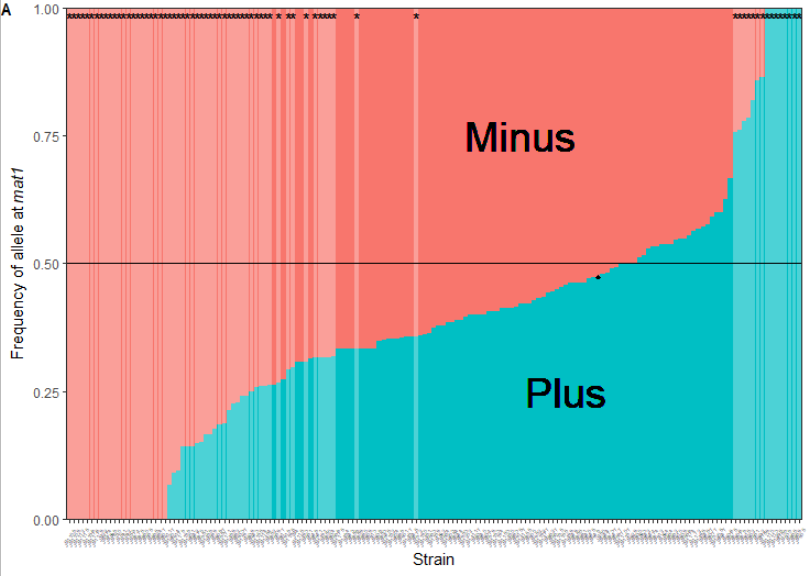image-20220624151532821

We also added a point for the reference lab strain (JB50), for which the actual value is 0.487

``````# show the value for data point JB50
ill_chi %>% ## add a point at the reference h90 strain.
filter( !str_detect( strainLong, "EBC")) %>%
mutate(strainLong = fct_reorder(strainLong, freq_mat1P_c)) %>%
mutate( point = ifelse(strainLong == "JB50", freq_mat1P_c,-1) ) %>%
filter( strainLong == "JB50" ) %>% select(strainLong, freq_allP_c)
# strainLong freq_allP_c
#   <fct>            <dbl>
#   JB50             0.487``````

To summarise these:

``````# Summarise the number of strains that deviate from 1:1
ill_chi %>%
filter( !str_detect( strainLong, "EBC")) %>%
filter( freq_mat1P_c != 0, freq_mat1P_c !=1   ) %>%
summarise( total = n(),
fdr = sum(!fdrtest),
MoreM = sum( freq_mat1P_c < 0.5 & !fdrtest),
MoreP = sum( freq_mat1P_c > 0.5 & !fdrtest))
#   # A tibble: 1 x 4
#     total   fdr MoreM MoreP
#     <int> <int> <int> <int>
#     131    41   34    7``````

Of the 161 strain, 131 strains are not heterothallic and of those, 41 are deviating. 7 have more P and 34 have more M reads at mat1.

To compare how the results at mat1 and at all loci (incl. mat1) are related, we can make this plot:

``````# Plot the mating type allele frequency at all loci
# Again, only for JB strains
FS2a <-
ill_chi %>%
mutate( freq_allM = 1 - freq_allP_c ) %>%      ## calculate the other half
mutate( ordervar = freq_allP_c ) %>%            ## make a sorting variable
pivot_longer( names_to = "mat",                  ## transform to longer
values_to = "freqs_all",
cols = starts_with("freq_all")) %>%
filter( !str_detect( strainLong, "EBC")) %>%   ## remove EBC strains
mutate(strainLong = fct_reorder(strainLong, ordervar)) %>%
mutate(signif = ifelse(fdrtestAll,"",no = "*")) %>%
mutate(sigcols = ifelse(fdrtestAll, 0, freqs_all ) ) %>%
ggplot( aes( x = strainLong, y =  freqs_all , fill = mat ) ) +
geom_bar( stat = "identity", width = 1) +
geom_bar( aes(x = strainLong, y = sigcols ), stat = "identity", fill = "white", alpha = 0.3) +
xlab("Strain") +
ylab(expression( "Frequency of allele at "*italic("mat1" ) ) ) +
scale_y_continuous(limits = c(0,1), expand = c(0, 0)) +
geom_hline( yintercept = 0.5) +
geom_text(x = 80, y=0.75, label="Minus", size=10) +  # add minus title
geom_text(x = 90, y=0.25, label="Plus", size=10)  + # add plus title
geom_hline( yintercept = 0.5) +
geom_text( aes( label= signif, y = 0.98)) + # add significance asterisk
theme_bw() +
theme( legend.position = "none" ) +
theme( axis.text.x = element_text( angle=60, hjust=1, size = 4 ) ) +
geom_point( data =   ill_chi %>% ## add a point at the reference h90 strain.
filter( !str_detect( strainLong, "EBC")) %>%
mutate(strainLong = fct_reorder(strainLong, freq_allP_c)) %>%
mutate( point = ifelse(strainLong == "JB50", freq_allP_c,-1) ),
aes(x = strainLong, y = point ), fill = 1, shape=18
)
FS2a <- annotate_figure(FS2a, fig.lab = "A", fig.lab.face = "bold")

FS2a
ggsave("../Manuscript/Fig_S2a.pdf" ,plot = FS2a, units = "cm", width = 20, height = 10)
``````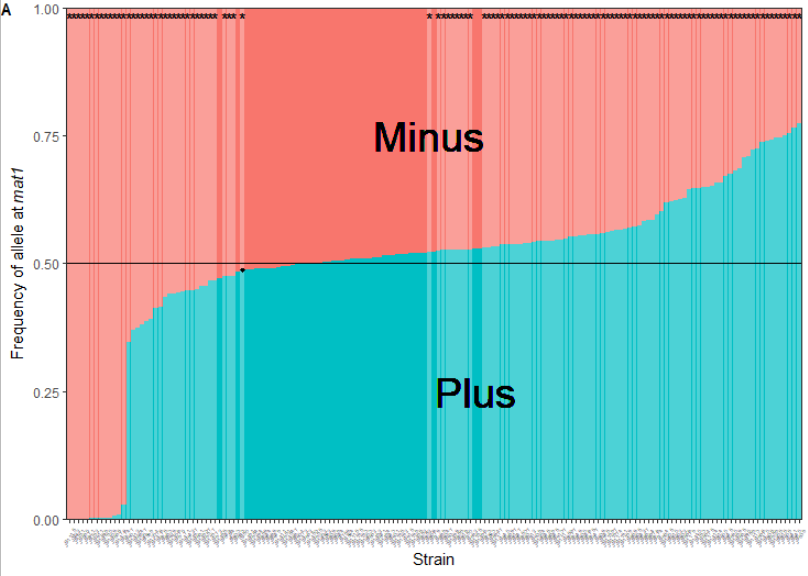image-20220624152720702

Many strains show more P alleles when considering all loci. We can plot this and the alleles at mat1 against each other:

``````### to check if there is a correlation between the total number of mat reads and
### the reads at mat1, this plots the freq P at both against each other,
### without EBC measurements.
FS2c <-
ill_chi %>%
filter( !str_detect( strainLong, "EBC")) %>%

ggplot(aes( x = freq_allP_c , y = freq_mat1P_c, size = mat1_reads_c)) +
geom_point() +
guides(size=guide_legend(title=expression("# "*italic("mat1P") *" reads") ) )+
xlab("Frequency of all P reads") +
ylab(expression( "Frequency of P reads at "*italic("mat1" ) ) ) +
scale_y_continuous(limits = c(0,1)) +
scale_x_continuous(limits = c(0,1)) +
geom_smooth( method = 'lm', size = 1, se = F) +
geom_smooth(data =
ill_chi %>%
mutate( freq_not_mat1_P =
) %>% filter( freq_not_mat1_P > 0.05, freq_not_mat1_P < 0.90)
,aes(freq_not_mat1_P,freq_mat1P_c),
method = 'lm', size = 1, se = F, color = 'red', linetype="longdash") +
theme_bw() +
theme( axis.text.x=element_text(angle=60,hjust=1))
FS2c <- annotate_figure(FS2c, fig.lab = "C", fig.lab.face = "bold")

FS2c
ggsave("../Manuscript/Fig_S2c.pdf" ,plot = FS2c, units = "cm", width = 13, height = 10)``````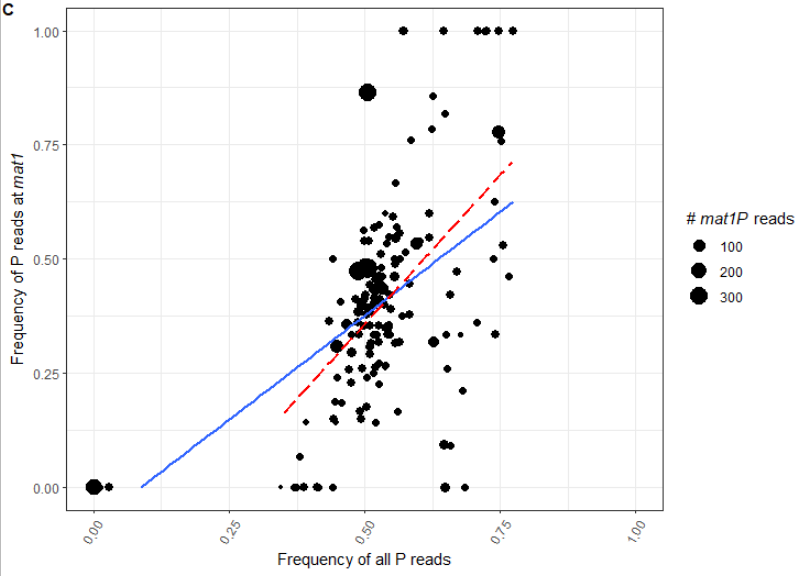image-20220624152948772

These results suggest a correlation between the number of P reads and the skew at mat1, however the overall number of reads is skewed to P (most data is to the right of 0.5). The lines show this with and without the presumed heterothallic strains and show strong significant correlations:

``````## test significance using binomal glm
cordata <- ill_chi %>%
mutate( freq_not_mat1_P =
)  %>% filter( !str_detect( strainLong, "EBC"))

mod_without_heterothallic <- glm( cbind(mat1P, mat1M ) ~ freq_allP_c , family = binomial(),
data= filter( cordata, freq_not_mat1_P > 0.05, freq_not_mat1_P < 0.90 ))
summary(mod_without_heterothallic)
plot(mod_without_heterothallic)
#Call:
#glm(formula = cbind(mat1P, mat1M) ~ freq_allP_c, family = binomial(),
#    data = filter(cordata, freq_not_mat1_P > 0.05, freq_not_mat1_P < 0.9))
#Deviance Residuals:
#    Min       1Q   Median       3Q      Max
#-9.5021  -1.7844  -0.3647   0.5767  16.0874
#
#Coefficients:
#            Estimate Std. Error z value Pr(>|z|)
#(Intercept)  -3.0266     0.1746  -17.33   <2e-16 ***
#freq_allP_c   5.1752     0.3188   16.23   <2e-16 ***
#---
#Signif. codes:  0 ‘***’ 0.001 ‘**’ 0.01 ‘*’ 0.05 ‘.’ 0.1 ‘ ’ 1
#
#(Dispersion parameter for binomial family taken to be 1)
#
#    Null deviance: 1623.7  on 147  degrees of freedom
#Residual deviance: 1341.4  on 146  degrees of freedom
#AIC: 1859.3
#Number of Fisher Scoring iterations: 4

mod_incl_heterothallic <- glm( cbind(mat1P, mat1M ) ~ freq_allP_c , family = binomial(),
data= cordata)
summary(mod_incl_heterothallic)
plot(mod_incl_heterothallic)
#Call:
#glm(formula = cbind(mat1P, mat1M) ~ freq_allP_c, family = binomial(),
#    data = cordata)
#
#Deviance Residuals:
#   Min      1Q  Median      3Q     Max
#-9.878  -1.641  -0.667   0.590  16.559
#
#Coefficients:
#            Estimate Std. Error z value Pr(>|z|)
#(Intercept)  -3.7175     0.1388  -26.78   <2e-16 ***
#freq_allP_c   6.4242     0.2558   25.12   <2e-16 ***
#---
#Signif. codes:  0 ‘***’ 0.001 ‘**’ 0.01 ‘*’ 0.05 ‘.’ 0.1 ‘ ’ 1
#
#(Dispersion parameter for binomial family taken to be 1)
#
#    Null deviance: 2607.8  on 160  degrees of freedom
#Residual deviance: 1401.4  on 159  degrees of freedom
#AIC: 1919.2
#Number of Fisher Scoring iterations: 5``````

One possibility might be that the skew in ‘all’ reads is associated with the copy number of the mating type cassettes, however, this does not seem to hold. We can test this using relative coverage of P and M cassettes (normalized by mean coverage outside of mating type), from Nieuwenhuis, Tusso et al. 2018.

``````#### Copies per region ####
col_types = "ffddddddddddddddddddddd") %>%
filter( sample_ID != "ERR499321" ) %>%
mutate( matP_freq = Mat_Plus_rel/(Mat_Minus_rel + Mat_Plus_rel ) )

# join this data with the data from the Illumina results
regions <-
inner_join( regions, filter( combined, mated == "mated" )  ) %>%
filter(strain != "JB22") %>%
mutate( mat1P = freq_mat1P * mat1_reads,
all_P = freq_allP * allmat_reads) %>%
group_by( strain ) %>%
summarize(  mat1P = sum(mat1P),
all_P = sum(all_P),
base_coverage = mean( base_coverage),
L_region   = mean( L_region_rel  ),
Mat_Minus   = mean( Mat_Minus_rel  ),
Mat_Plus   = mean( Mat_Plus_rel  ),
K_region   = mean( K_region_rel  ),
IR_regions    = mean( IR_regions_rel   )
) %>%

FS2b<-
regions %>%
ggplot( aes(x = Mat_Plus, y = K_region, size = allmat_reads)) +
geom_smooth( method = "lm", show.legend = F, size = 1, se = F  ) +
geom_point() +
ylab(expression( "Relative coverage of "*italic("K" )*"-region" ) ) +
xlab(expression( "Relative coverage of P cassette" ) ) +
guides(size=guide_legend(title=expression( "# "*italic("P" )*" reads" ))) +
theme_bw()

FS2b <- annotate_figure(FS2b, fig.lab = "B", fig.lab.face = "bold")

FS2b
ggsave("../Manuscript/Fig_S2b.pdf" ,plot = FS2b, units = "cm", width = 13, height = 10)
``````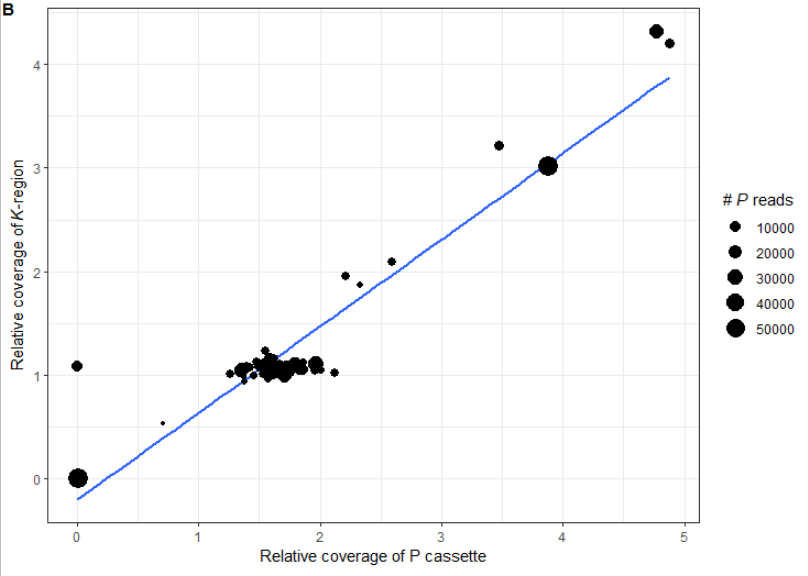image-20220624153459129

This shows that the relative coverage of the Plus cassette is closely associated with the K region, suggesting repeats of the entire silent mating type region. This is not the case for the Minus cassettes, which generally is not duplicated when the silent region is duplicated.

``````regions %>%
ggplot( aes(x = Mat_Minus, y = K_region, size = allmat_reads)) +
geom_smooth( method = "lm", show.legend = F, size = 1, se = F  ) +
geom_point() +
ylab(expression( "Relative coverage of "*italic("K" )*"-region" ) ) +
xlab(expression( "Relative coverage of M cassette" ) ) +
guides(size=guide_legend(title=expression( "# "*italic("P" )*" reads" ))) +
theme_bw()``````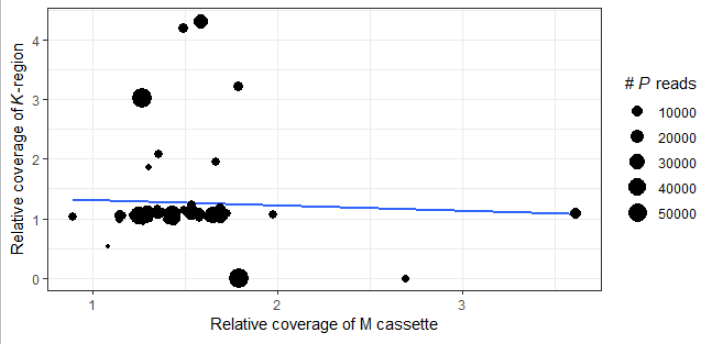image-20220628185806029

Finally, comparing the relative coverage at P for all loci with the allele frequency of P at mat1 shows that the latter has a large range of frequencies, from 0 to 1, and within the expected 1.5 coverage (‘wild type’ copy number with P at mat2 and sometimes at mat1) though smaller, the range still goes from ~0.15 to 0.9.

``````#### Fig 2c - all v mat1 ####

F2c <-
regions %>%
ggplot( aes(y = freq_mat1P, x = Mat_Plus, size = allmat_reads)) +
geom_point() +
ylab(expression( "Frequency of P at "*italic("mat1" ) ) ) +
xlab(expression(  "Relative coverage of P at all loci" ) ) +
guides(size=guide_legend(title=expression( "# "*italic("P" )*" reads" ))) +
theme_bw()
F2c <- annotate_figure(F2c, fig.lab = "C", fig.lab.face = "bold")

F2c
ggsave("../Manuscript/Fig_2c.pdf" ,plot = F2c, units = "cm", width = 13, height = 10)
``````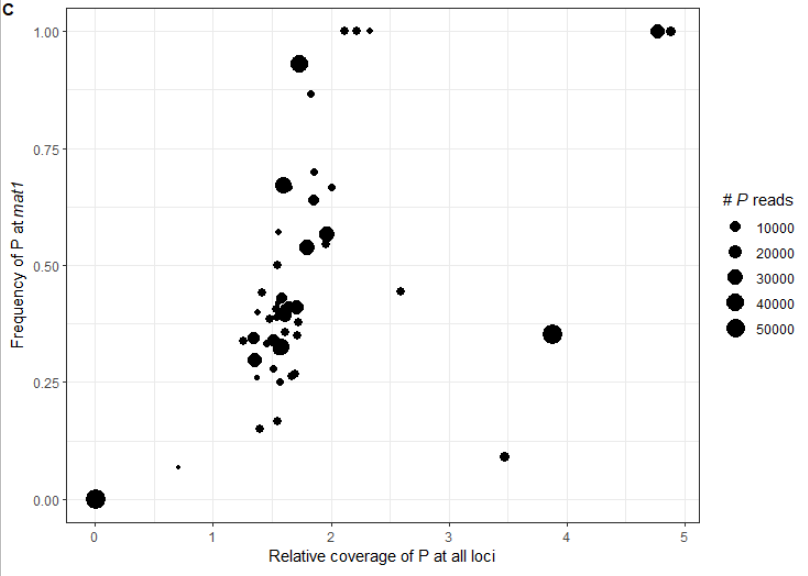image-20220628185709614

### Verify RTS1 region

We performed a check to see if the RTS1 regions is a single copy region by comparing coverage of this region to the mean coverage of the strain (see script above). We aggregate all values per strain into one file:

``````# when all individual files are generated, we can analyse the results using this script
for F in *.coverage; do
awk '{ total += \$3 } END { if(NR>0) print FILENAME total/NR; else print FILENAME " 0" }' \$F >> coverage.txt
done``````

And visualize this in R using:

``````library(tidyverse)
#### Coverage for RTS1 region ####

cover <- read_delim( "data/coverages/coverage.txt", col_types = "fn", delim = " ", col_names = F )
names(cover) <- c("strain", "coverage")
cover <- cover %>%
separate( strain, into = c("strain", "accession", "ref","region"),extra = "drop" ) %>%
mutate( region = ifelse( region == "coverage", "II100kb", region ) )

mean_cover <- cover %>% group_by(strain, ref, region) %>%
summarize( m_cover = mean(coverage)) %>%
ungroup() %>%
mutate( strain = as.factor(strain)) %>%
pivot_wider(values_from = m_cover, names_from = "region" )

#### Fig S1 RTS1 ####
FS1 <-
cover %>%
group_by(strain, region) %>%
summarize( m_cover = mean(coverage)) %>%
ungroup() %>%
mutate( strain = as.factor(strain)) %>%
pivot_wider(values_from = m_cover, names_from = "region" ) %>%
ggplot(aes(x = strain, y = RTS1/II100kb )) +
geom_point() +
ylim(0,1.2) +
theme_bw() +
ylab(expression( "Normalized coverage at "*italic("RTS1" )*" region" ) ) +
xlab("Strain") +
ggtitle('Fig. S1') +
theme( axis.text.x=element_text(angle=60,hjust=1, size = 5),
panel.grid.major.x = element_blank(),
plot.title = element_text(hjust = -0.08, vjust=0.12))

FS1
ggsave("../Manuscript/Fig_S1.pdf", plot = FS1, units = "cm", width = 20, height = 10)``````